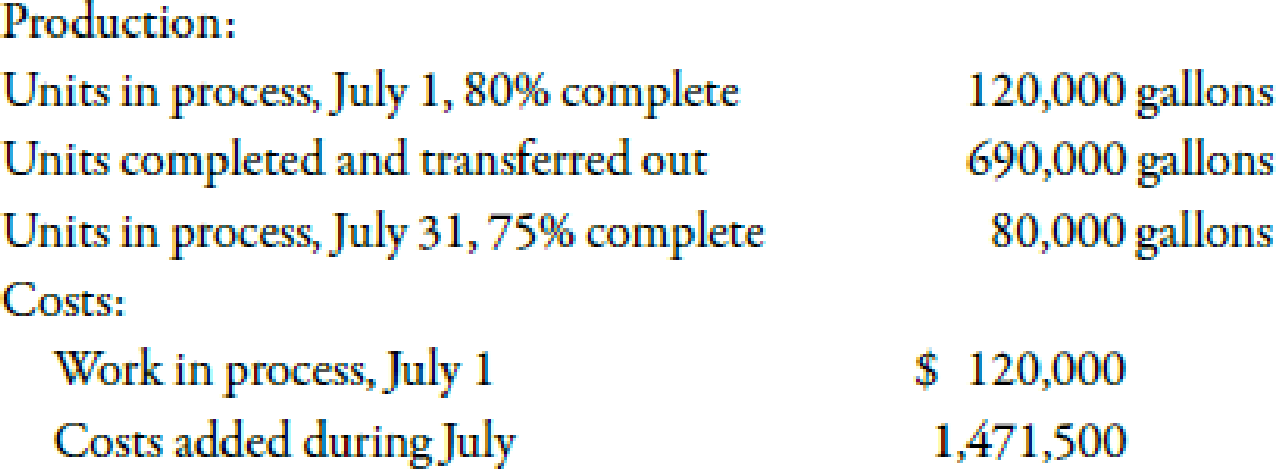# Use the following information for Brief Exercises 6-29 and 6-30: Aztec Inc. produces soft drinks. Mixing is the first department, and its output is measured in gallons. Aztec uses the FIFO method. All manufacturing costs are added uniformly. For July, the mixing department provided the following information: 6-29 (Appendix 6A) First-In, First-Out Method; Equivalent Units Refer to the information for Aztec Inc. on the previous page. Required: 1. Calculate the equivalent units for July. 2. Calculate the unit cost. ( Note: Round to two decimal places.) 3. Assign costs to units transferred out and EWIP using the FIFO method.### Managerial Accounting: The Corners...

7th Edition
Maryanne M. Mowen + 2 others
Publisher: Cengage Learning
ISBN: 9781337115773### Managerial Accounting: The Corners...

7th Edition
Maryanne M. Mowen + 2 others
Publisher: Cengage Learning
ISBN: 9781337115773

#### Solutions

Chapter
Section
Chapter 6, Problem 29BEA
Textbook Problem
250 views

## Use the following information for Brief Exercises 6-29 and 6-30:Aztec Inc. produces soft drinks. Mixing is the first department, and its output is measured in gallons. Aztec uses the FIFO method. All manufacturing costs are added uniformly. For July, the mixing department provided the following information:6-29 (Appendix 6A) First-In, First-Out Method; Equivalent UnitsRefer to the information for Aztec Inc. on the previous page.Required: 1. Calculate the equivalent units for July. 2. Calculate the unit cost. (Note: Round to two decimal places.) 3. Assign costs to units transferred out and EWIP using the FIFO method.

Expert Solution

1.

To determine

Compute equivalent units for July.

### Explanation of Solution

Equivalent Units of Production:

The total units of finished goods along with those goods that are not fully complete are known as equivalent units of production. In other words, equivalent units of production measures both completed and nearly completed units.

Use the following formula to calculate the equivalent units:

Equivalent units=(Units started and completed+Units in BWIP+Units in EWIP

Expert Solution

2.

To determine

Compute the unit cost of Company SI.

Expert Solution

3.

To determine

Calculate cost of units transferred-out and cost of EWIP by using FIFO method.

### Want to see the full answer?

Check out a sample textbook solution.See solution

### Want to see this answer and more?

Bartleby provides explanations to thousands of textbook problems written by our experts, many with advanced degrees!

See solution